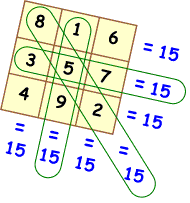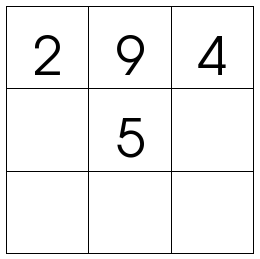# Maths Rotations: Magic Addition Squares

For maths today we are going to use magic addition squares. A magic addition  square is a square with 3 rows and 3 columns. Each grid of the square has to have a number in it and every line (horizontal, vertical and diagonal needs to add up to the same number – the magic number). Below are two images of a magic addition squares. One of the squares is complete and the other is incomplete. We will work out the incomplete square together.When we complete magic addition squares we need to use our addition strategies to assist us in adding the numbers together.

At the end of the session we will talk about the addition strategies that we were able to use.